# NCERT Solutions For Class 11 Maths Chapter 6 Exercise 6.3

## Chapter 6 Ex.6.3 Question 1

Solve the following system of inequality graphically: $$x \ge 3,y \ge 2.$$

### Solution

\begin{align}&x \ge 3\;\;\;\;\;\;\;\;\;\;\;\;\;\; \ldots \left( 1 \right)\\&y \ge 2\;\;\;\;\;\;\;\;\;\;\;\;\; \ldots \left( 2 \right)\end{align}

The graph of the lines, $$x = 2$$ and $$y = 2,$$ are drawn in the figure below.

Inequality $$x \ge 3$$ represents the region on the right hand side of the line, $$x = 2$$ (including the line $$x = 2$$), and inequality $$y \ge 2$$ represents the region above the line, $$y = 2$$ (including the line $$y = 2$$).

Hence, the solution of the given system of linear inequalities is represented by the common shaded region including the points on the respective lines as follow: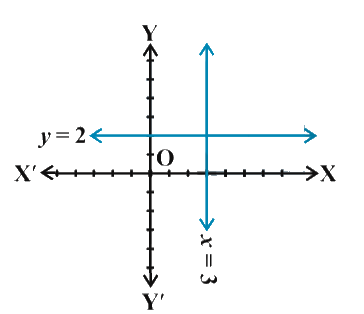## Chapter 6 Ex.6.3 Question 2

Solve the following system of inequalities graphically: $$3x + 2y \le 12,x \ge 1,y \ge 2.$$

### Solution

\begin{align}&3x + 2y \le 12\;\;\;\;\;\;\; \ldots \left( 1 \right)\\&x \ge 1\;\;\;\;\;\;\;\;\;\;\;\;\;\;\; \ldots \left( 2 \right)\\&y \ge 2\;\;\;\;\;\;\;\;\;\;\;\;\;\;\; \ldots \left( 3 \right)\end{align}

The graphs of the lines, $$3x + 2y = 12,x = 1,$$ and $$y = 2,$$ are drawn in the figure below.

Inequality $$3x + 2y \le 12$$represents the region below the line $$3x + 2y = 12$$ (including the line $$3x + 2y = 12$$).

Inequality $$x \ge 1$$ represents the region on the right hand side of the line , (including the line $$x = 1$$).

Inequality $$y \ge 2$$ represents the region above the line, $$y = 2$$ (including the line $$y = 2$$).

Hence, the solution of the given system of linear inequalities is represented by the common shaded region including the points on the respective lines as follows: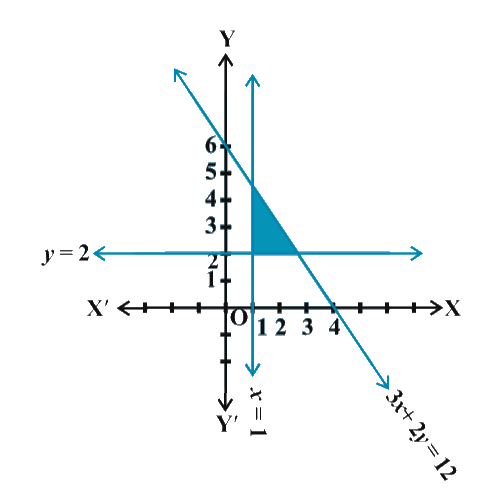## Chapter 6 Ex.6.3 Question 3

Solve the following system of inequalities graphically: $$2x + y \ge 6,3x + 4y$$$$\le 12.$$

### Solution

\begin{align}&2x + y \ge 6\;\;\;\;\;\;\;\;\;\;\;\;\;\;\;\;\;\, \ldots \left( 1 \right)\\&3x + 4y \le 12\;\;\;\;\;\;\;\;\;\;\;\;\;\; \ldots \left( 2 \right)\end{align}

The graphs of the lines, $$2x + y = 6$$ and $$3x + 4y = 12$$ are drawn in the figure below.

Inequality $$2x + y \ge 6$$ represents the region below the line, $$2x + y = 6$$ (including the line $$2x + y = 6$$ ).

Inequality $$3x + 4y \le 12$$ represents the region on the right hand side of the line, $$3x + 4y = 12$$ (including the line $$3x + 4y = 12$$).

Hence, the solution of the given system of linear inequalities is represented by the common shaded region including the points on the respective lines as follows: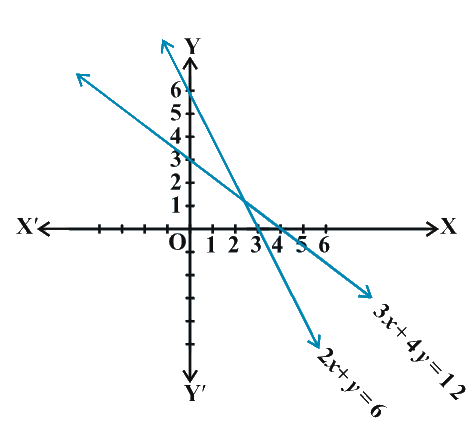## Chapter 6 Ex.6.3 Question 4

Solve the following system of inequalities graphically: $$x + y \ge 4,2x - y \lt 0.$$

### Solution

\begin{align} & x+y\ge 4\ \ \ \ \ \ \ \ \ \ \ \ \ \ \ldots \left( 1 \right) \\ & 2x-y\lt0\ \ \ \ \ \ \ \ \ \ \ \ \ \ \ldots \left( 2 \right) \end{align}

The graphs of the lines,$$x + y = 4$$and $$2x - y = 0$$ are drawn in the figure below.

Inequality $$x + y \ge 4$$ represents the region above the line, $$x + y = 4$$ (including the line $$x + y = 4$$).

It is observed that $$\left( {{\rm{1}},0} \right)$$ satisfies the inequality, $$2x - y \lt 0$$. Since, $$2\left( 1 \right) - 0 = 2 \lt 0$$

Therefore, Inequality $$2x - y \lt 0$$ represents the half plane corresponding to the line , $$2x - y = 0$$, containing the point (1,0) (excluding the line $$2x - y \lt 0$$).

Hence, the solution of the given system of linear inequalities is represented by the common shaded region including the points on the line $$x + y = 4$$ and excluding the points on line $$2x - y = 0$$ as follows.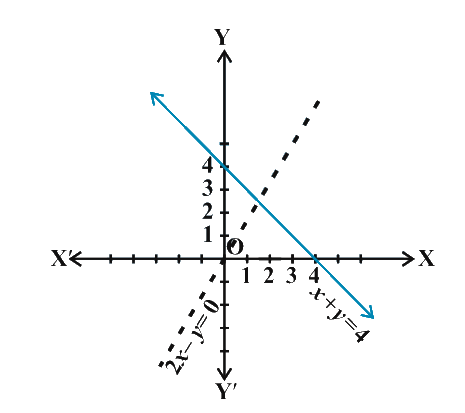## Chapter 6 Ex.6.3 Question 5

Solve the following system of inequalities graphically: $$2x - y \gt 1,x - 2y \lt - 1$$

### Solution

\begin{align} & 2x-y\gt1\ \ \ \ \ \ \ \ \ \ \ \ \ \ \ \ \ldots \left( 1 \right) \\ & x-2y\lt-1\ \ \ \ \ \ \ \ \ \ \ \ \ \ldots \left( 2 \right) \end{align}

The graphs of the lines, $$2x - y = 1$$and $$x - 2y = - 1$$ are drawn in the figure below.

Inequality $$2x - y \gt 1$$ represents the region below the line, $$2x - y = 1$$(excluding the line $$2x - y = 1$$).

Inequality $$x - 2y \lt - 1$$ represents the region on the right hand side of the line, $$x - 2y = - 1$$ (excluding the line $$x - 2y = - 1$$).

Hence, the solution of the given system of linear inequalities is represented by the common shaded region excluding the points on the respective lines as follows: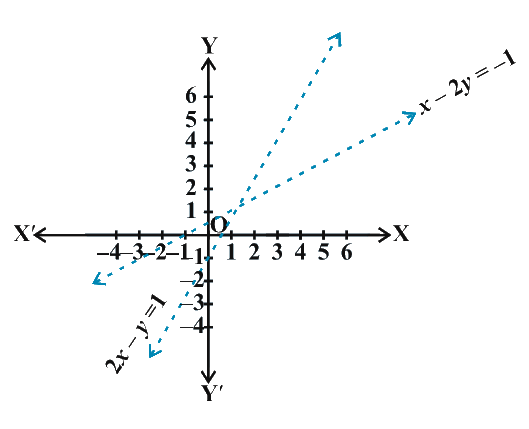## Chapter 6 Ex.6.3 Question 6

Solve the following system of inequalities graphically: $$x + y \le 6,x + y \ge$$ $$x + y = 6$$4

### Solution

\begin{align}&x + y \le 6\;\;\;\;\;\;\;\;\;\;\;\;\;\;\;\; \ldots \left( 1 \right)\\&x + y \ge 4\;\;\;\;\;\;\;\;\;\;\;\;\;\;\;\; \ldots \left( 2 \right)\end{align}

The graphs of the lines, $$x + y = 6$$ and $$x + y = 4$$ are drawn in the figure below.

Inequality $$x + y \le 6$$ represents the region below the line, (including the line $$x + y = 6$$).

Inequality $$x + y \ge 4$$ represents the region on the right hand side of the line, $$x + y = 4$$ (including the line $$x + y = 4$$).

Hence, the solution of the given system of linear inequalities is represented by the common shaded region including the points on the respective lines as follows: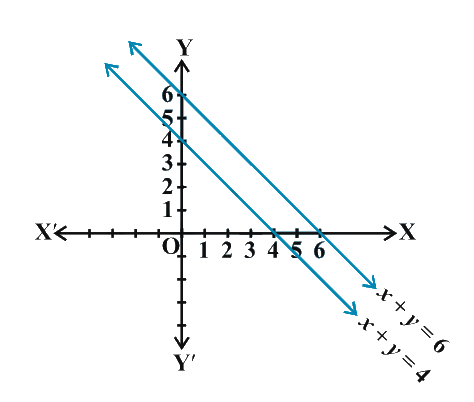## Chapter 6 Ex.6.3 Question 7

Solve the following system of inequalities graphically: $$2x + y \ge 8,x + 2y \ge 10$$

### Solution

\begin{align}&2x + y \ge 8\;\;\;\;\;\;\;\;\;\;\;\;\;\;\;\; \ldots \left( 1 \right)\\&x + 2y \ge 10\;\;\;\;\;\;\;\;\;\;\;\;\;\; \ldots \left( 2 \right)\end{align}

The graphs of the lines, $$2x + y = 8$$ and $$x + 2y = 10$$ are drawn in the figure below.

Inequality $$2x + y \ge 8$$ represents the region below the line, $$2x + y = 8$$ (including the line).

Inequality $$x + 2y \ge 10$$ represents the region on the right hand side of the line, $$x + 2y = 10$$ (including the line).

Hence, the solution of the given system of linear inequalities is represented by the common shaded region including the points on the respective lines as follows: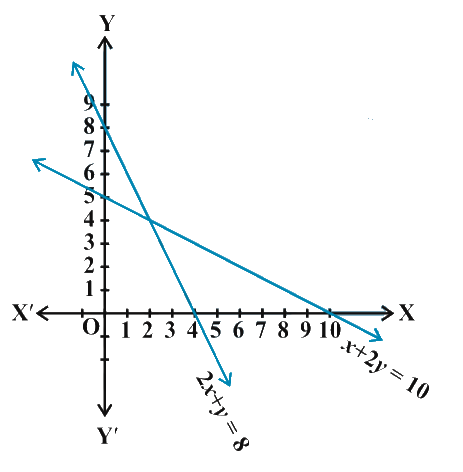## Chapter 6 Ex.6.3 Question 8

Solve the following system of the inequalities graphically: $$x + y \le 9, y \gt x, x \ge 0$$

### Solution

\begin{align}&x + y \le 9\;\;\;\;\;\;\;\;\;\; \ldots \left( 1 \right)\\&y \gt x\;\;\;\;\;\;\;\;\;\;\;\;\;\;\;\; \ldots \left( 2 \right)\\&x \ge 0\;\;\;\;\;\;\;\;\;\;\;\;\;\;\;\; \ldots \left( 3 \right)\end{align}

The graph of the lines, $$x + y = 9$$ and $$y = x$$, are drawn in the figure below.

Inequality $$x + y \le 9$$ represents the region below the line, $$x + y = 9$$ (including the line $$x + y = 9$$).

It is observed that $$\left( {0,{\rm{1}}} \right)$$ satisfies the inequality,$$y = x$$. Since, $$1 \gt 0$$.

Therefore, inequality $$y \gt x$$ represents the half plane, corresponding to the line, $$y = x$$, containing the point $$\left( {0,{\rm{1}}} \right)$$, (excluding the line $$y = x$$).

Inequality $$x \ge 0$$ represents the region on the right hand side of the line, $$x = 0$$ or y-axis (including the y-axis).

Hence, the solution of the given system of linear inequalities is represented by the common shaded region including the points on the lines, $$x + y = 9$$ and $$x = 0$$, but excluding the points on line $$y = x$$ as follows: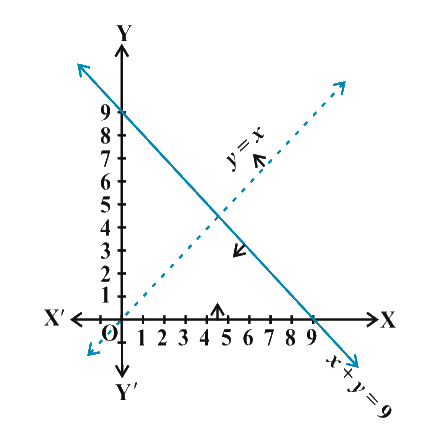## Chapter 6 Ex.6.3 Question 9

Solve the following system of inequalities graphically: $$5x + 4y \le 20,\;x \ge 1,\; y \ge 2$$

### Solution

\begin{align}&5x + 4y \le 20 \;\;\qquad \ldots \left( 1 \right)\\&x \ge 1 \qquad\qquad\qquad \ldots \left( 2 \right)\\ &y \ge 2 \qquad\qquad\qquad \ldots \left( 3 \right)\end{align}

The graph of the lines, $$5x + 4y = 20$$, $$x = 1$$ and $$y = 2$$, are drawn in the figure below.

Inequality $$5x + 4y \le 20$$ represents the region below the line, $$5x + 4y = 20$$ (including the line $$5x + 4y = 20$$).

Inequality $$x \ge 1$$ represents the region below the line, $$x = 1$$ (including the line $$x = 1$$).

Inequality $$y \ge 2$$ represents the region below the line, $$y = 2$$ (including the line $$y = 2$$).

Hence, the solution of the given system of linear inequalities is represented by the common shaded region including the points on the respective lines as follows: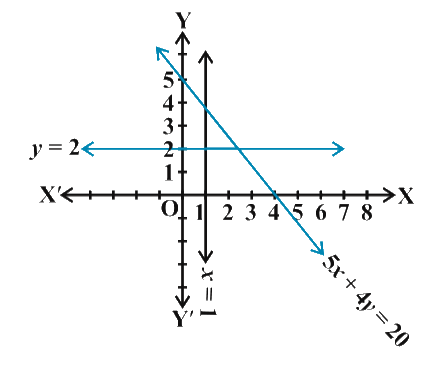## Chapter 6 Ex.6.3 Question 10

Solve the following system of inequalities graphically: $$3x + 4y \le 60,\;x + 3y \le 30,\;x \ge 0,\;y \ge 0$$

### Solution

\begin{align}&3x + 4y \le 60 \qquad\ldots \left( 1 \right)\\&\;\;x + 3y \le 30 \qquad \ldots \left( 2 \right)\end{align}

The graph of the lines, $$3x + 4y = 60$$ and $$x + 3y = 30$$, are drawn in the figure below.

Inequality $$3x + 4y \le 60$$ represents the region below the line, $$3x + 4y = 60$$ (including the line $$3x + 4y = 60$$).

Inequality $$x + 3y \le 30$$ represents the region the line, $$x + 3y = 30$$ (including the line $$x + 3y = 30$$).

Since $$x \ge 0$$ and $$y \ge 0$$ every point in the common shaded region in the first quadrant including the points on the respective line and the axes represents the solution of the given system of linear inequalities as follows: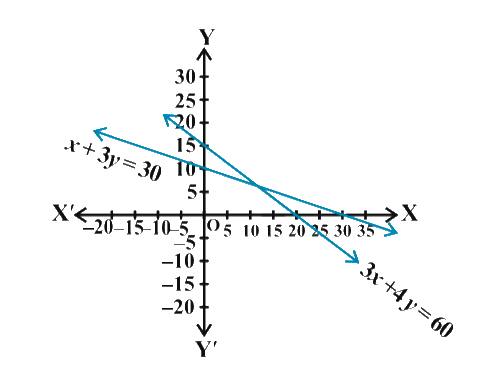## Chapter 6 Ex.6.3 Question 11

Solve the following system of inequalities graphically: $$2x + y \ge 4,\;x + y \le 3,\;2x - 3y \le 6$$

### Solution

\begin{align}&\;2x + y \ge 4\;\;\;\;\;\;\;\;\;\;\;\;\;\; \ldots \left( 1 \right)\\&\;\;x + y \le 3\;\;\;\;\;\;\;\;\;\;\;\;\;\;\; \ldots \left( 2 \right)\\&2x - 3y \le 6\;\;\;\;\;\;\;\;\;\;\;\;\; \ldots \left( 3 \right)\end{align}

The graph of the lines, $$2x + y = 4$$, $$x + y = 3$$ and $$2x - 3y = 6$$, are drawn in the figure below.

Inequality $$2x + y \ge 4$$ represents the region above the line, $$2x + y = 4$$ (including the line).

Inequality $$x + y \le 3$$ represents the region below the line, $$x + y = 3$$ (including the line).

Inequality $$2x - 3y \le 6$$ represents the region above the line, $$2x - 3y = 6$$ (including the line).

Hence, the solution of the given system of linear inequalities is represented by the common shaded region including the points on the respective lines as follows: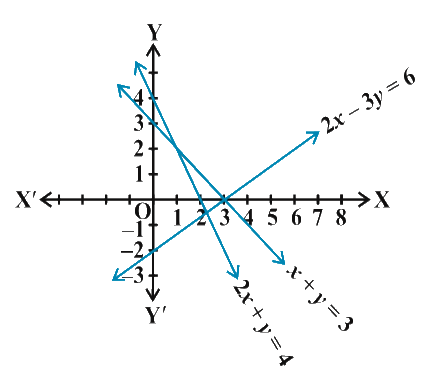## Chapter 6 Ex.6.3 Question 12

Solve the following system of inequalities graphically:

$x - 2y \le 3,\;3x + 4y \ge 12,\;x \ge 0,\;y \ge 1$

### Solution

\begin{align}&\;x - 2y \le 3\;\;\;\;\;\;\;\;\;\;\;\;\;\;\;\; \ldots \left( 1 \right)\\&3x + 4y \ge 12\;\;\;\;\;\;\;\;\;\;\;\;\; \ldots \left( 2 \right)\\&\quad y \ge 1\;\;\;\;\;\;\;\;\;\;\;\;\;\;\;\;\;\;\quad \ldots \left( 3 \right)\\&\quad x \ge 0\;\;\;\;\;\;\;\;\;\;\;\;\;\;\;\;\;\;\quad \ldots \left( 4 \right)\end{align}

The graphs of the lines, $$x - 2y = 3$$, $$3x + 4y = 12$$ and $$y = 1$$ are drawn in the figure below.

Inequality $$x - 2y \le 3$$ represents the region above the line, $$x - 2y = 3$$ (including the line).

Inequality $$3x + 4y \ge 12$$ represents region above the line, $$3x + 4y = 12$$ (including the line).

Inequality $$y \ge 1$$ represents the region above the line, $$y = 1$$ (including the line).

Inequality $$x \ge 0$$ represents the region on the right hand side of $$y-$$axis (including y-axis).

Hence, the solution of the given system of linear inequalities is represented by the common shaded region including the points on the respective lines and $$y-$$axis as follows: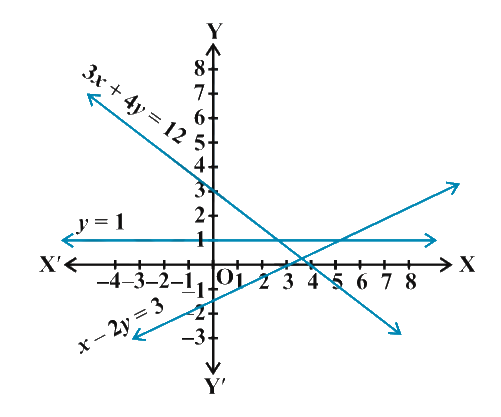## Chapter 6 Ex.6.3 Question 13

Solve the following system of inequalities graphically:

$4x + 3y \le 60,\;y \ge 2x,\;x \ge 3,\;x,y \ge 0$

### Solution

\begin{align}&4x + 3y \le 60\;\;\;\;\;\;\;\;\; \ldots \left( 1 \right)\\&\quad y \ge 2x\;\;\;\;\;\;\;\;\;\;\;\;\;\;\; \ldots \left( 2 \right)\\&\quad x \ge 3\;\;\;\;\;\;\;\;\;\;\;\;\;\;\;\;\; \ldots \left( 3 \right)\end{align}

The graph of the lines, $$4x + 3y = 60$$, $$y = 2x$$, and $$x = 3$$, are drawn in the figure below.

Inequality $$4x + 3y \le 60$$ represents the region below the line, $$4x + 3y = 60$$ (including the line $$4x + 3y = 60$$).

Inequality $$y \ge 2x$$ represents the region above the line, $$y = 2x$$ (including the line $$y = 2x$$).

Inequality $$x \ge 3$$ represents the region on the right hand side of the line, $$x = 3$$ (including the line$$x = 3$$).

Hence, the solution of the given system of linear inequalities is represented by the common shaded region including the points on the respective lines as follows: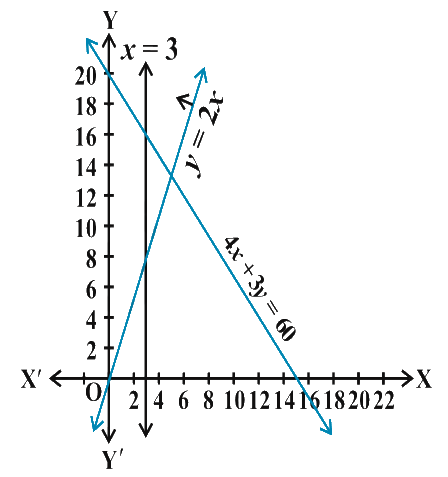## Chapter 6 Ex.6.3 Question 14

Solve the following system of inequalities graphically: $3x + 2y \le 150,\;x + 4y \le 80,\;x \le 15,\;y \ge 0,\;x \ge 0$

### Solution

\begin{align} & 3x+2y\le 150 \qquad \ldots \left( 1 \right) \\& \;x+4y\le 80\qquad\;\; \ldots \left( 2 \right) \\ & \quad x\le 15\qquad\quad\quad \ldots \left( 3 \right) \end{align}

The graph of the lines $$3x + 2y = 150$$, $$x + 4y = 80$$ and $$x = 15$$, are drawn in the figure below.

Inequality $$3x + 2y \le 150$$ represents the region below the line, $$3x + 2y = 150$$ (including the line $$3x + 2y = 150$$).

Inequality $$x + 4y \le 80$$ represents the region below the line, $$x + 4y = 80$$ (including the line $$x + 4y = 80$$).

Inequality $$x \le 15$$ represents the region on the left hand side the line, $$x = 15$$ (including the line $$x = 15$$).

Since $$x \ge 0$$ and $$y \ge 0$$, every point in the common shaded region in the first quadrant including the points on the respective lines and the axes represents the solution of the given system of linear inequalities as follows: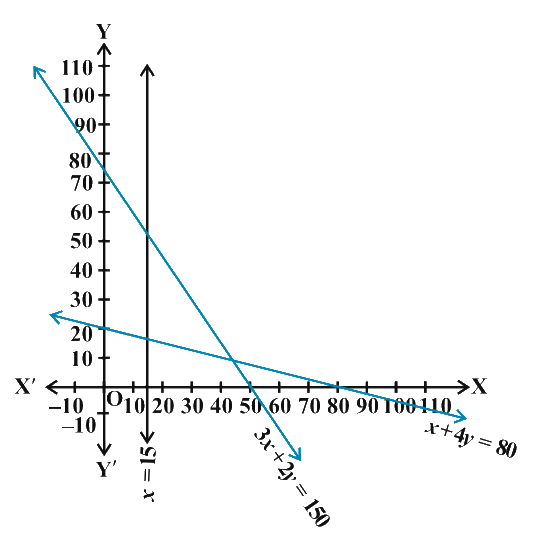## Chapter 6 Ex.6.3 Question 15

Solve the following system of inequalities graphically: $x + 2y \le 10,\;x + y \ge 1,\;x - y \le 0,\;x \ge 0,\;y \ge 0$

### Solution

\begin{align}&x + 2y \le 10\;\;\;\;\;\;\;\;\;\;\; \ldots \left( 1 \right)\\&x + y \ge 1\;\;\;\;\;\;\;\;\;\;\;\;\;\; \ldots \left( 2 \right)\\&x - y \le 0\;\;\;\;\;\;\;\;\;\;\;\;\;\; \ldots \left( 3 \right)\end{align}

The graph of the lines, $$x + 2y = 10$$, $$x + y = 1$$ and $$x - y = 0$$ are drawn in the figure below.

Inequality $$x + 2y \le 10$$ represents the region below the line, $$x + 2y = 10$$ (including the line $$x + 2y = 10$$).

Inequality $$x + y \ge 1$$ represents the region above the line, $$x + y = 1$$ (including the line $$x + y = 1$$).

Inequality (3) represents the region above the line, $$x - y = 0$$ (including the line $$x - y = 0$$).

Since $$x \ge 0$$ and $$y \ge 0$$ , every point in the common shaded region in the first quadrant including the points on the respective lines and the axes represents the solution of the given system of linear inequalities as follows: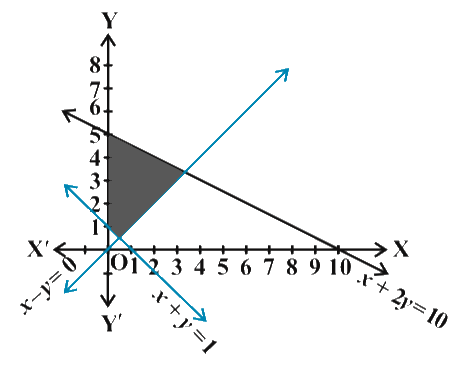Instant doubt clearing with Cuemath Advanced Math Program